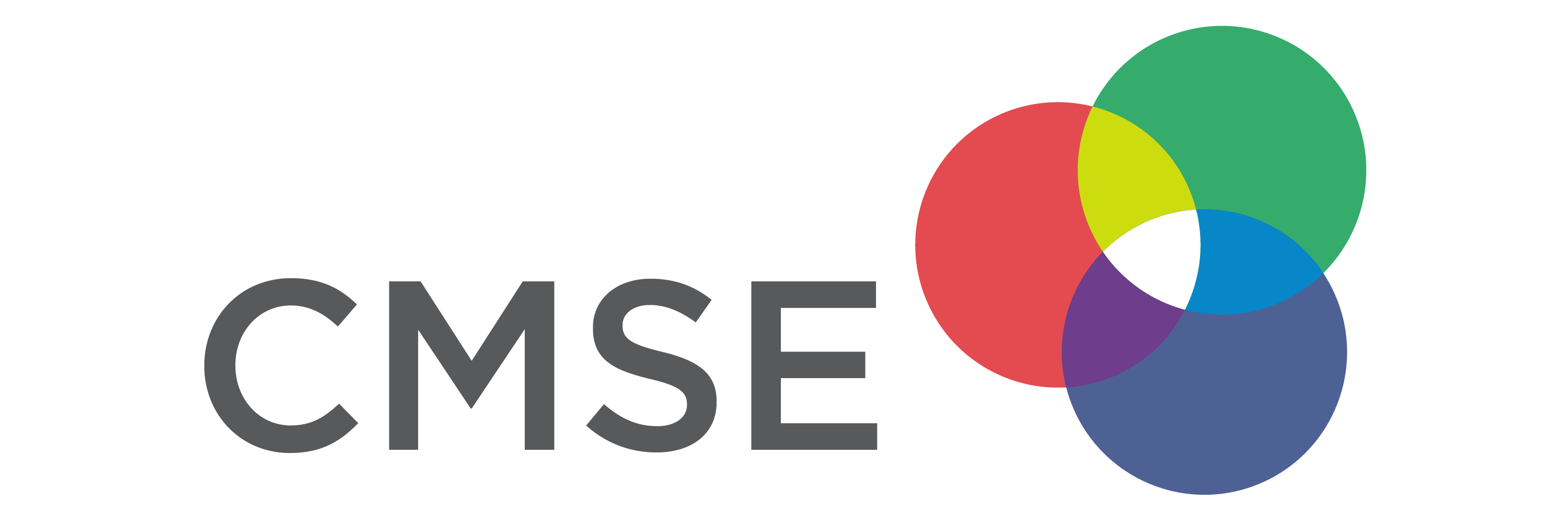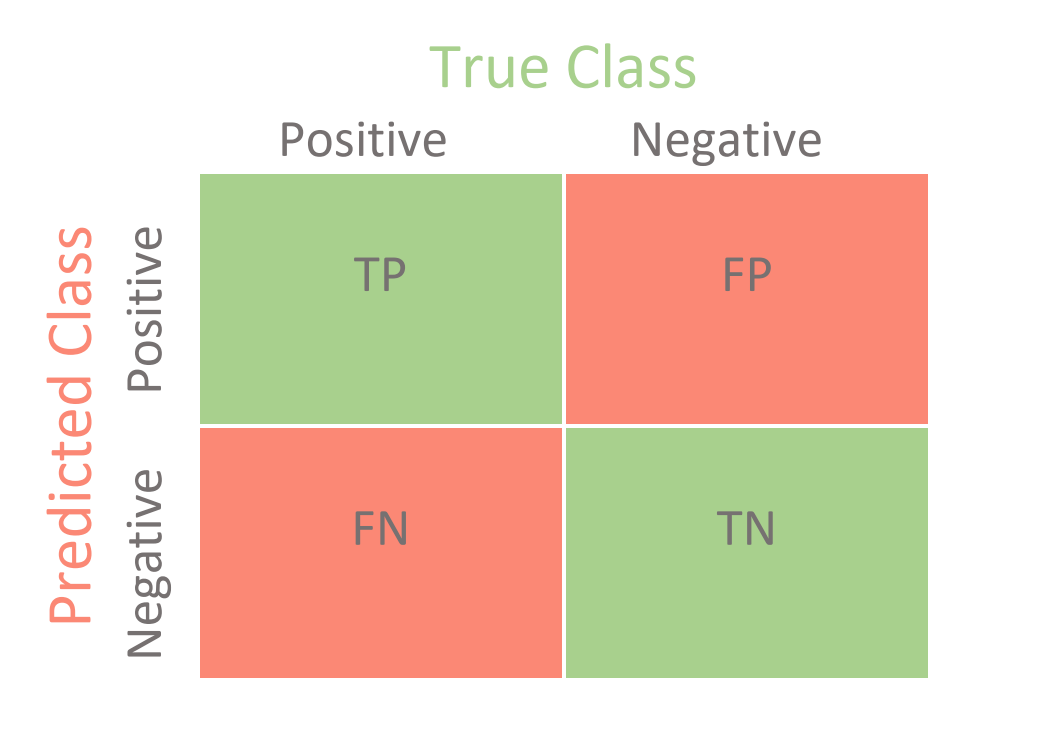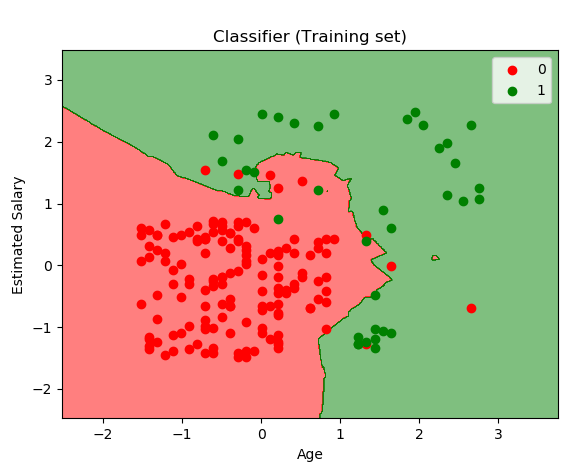# Day 10 - K Nearest Neighbors and Evaluating Classification Models¶

## Oct. 8, 2020¶# From Pre-Class Assignment¶

## Useful Stuff¶

• Videos were useful, but they were a little long
• I have a better idea of how we are evaluating classification models

## Challenging bits¶

• There's so much terminology, do I have to remember it all?
• I'm still confused about the ROC and what it is doing.
• How is KNN a binary classifier?

# The Confusion Matrix¶from sklearn.metrics import confusion_matrix
tn, fp, fn, tp = confusion_matrix(y_true, y_predicted)

Note the rows and columns of the confusion matrix from sklearn do not match those show on most websites.

# Other Metrics¶

• Sensitivity (Recall): The ratio of True Positives to all Positive Cases $\dfrac{TP}{TP+FN}$
• Specificity: The ratio of True Negatives to all Negative Cases $\dfrac{TN}{TN+FP}$
• Precision: The ratio of True Positives to all Predicted Positives: $\dfrac{TP}{TP+FP}$

• $F_1$ Score: A balanced measure (0 to 1) that includes sensitity and recall: $\dfrac{2 TP}{2TP + FP + FN}$

# ROC Curve and AUC¶from sklearn import metrics
fpr, tpr, thresholds = metrics.roc_curve(y_true, y_predict)
roc_auc = metrics.auc(fpr, tpr)
plt.plot(fpr, tpr)

# KNN as a Binary Classifier¶## A Heads Up for Today¶

Working with Pima Diabetes Database, which has problems (zeros for various entries). We have given you a cleaned data set on D2L (you will need to download it again!).

You can skip 2.1 and 2.2 today and go to 2.3; we will discuss how to clean that data after class.